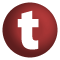# Sorted

#### trait Sorted[K, +This <: Sorted[K, This]] extends AnyRef

Any collection (including maps) whose keys (or elements) are ordered.

known subclasses: SortedSetLike, SortedMapLike

1. AnyRef
2. Any

### Value Members

1. #### def compare(k0: K, k1: K): Int

Comparison function that orders keys

Comparison function that orders keys.

2. #### def equals(arg0: Any): Boolean

This method is used to compare the receiver object (`this`) with the argument object (`arg0`) for equivalence

This method is used to compare the receiver object (`this`) with the argument object (`arg0`) for equivalence.

The default implementations of this method is an equivalence relation:

• It is reflexive: for any instance `x` of type `Any`, `x.equals(x)` should return `true`.
• It is symmetric: for any instances `x` and `y` of type `Any`, `x.equals(y)` should return `true` if and only if `y.equals(x)` returns `true`.
• It is transitive: for any instances `x`, `y`, and `z` of type `AnyRef` if `x.equals(y)` returns `true` and `y.equals(z)` returns `true`, then `x.equals(z)` should return `true`.

If you override this method, you should verify that your implementation remains an equivalence relation. Additionally, when overriding this method it is often necessary to override `hashCode` to ensure that objects that are "equal" (`o1.equals(o2)` returns `true`) hash to the same `Int` (`o1.hashCode.equals(o2.hashCode)`).

arg0

the object to compare against this object for equality.

returns

`true` if the receiver object is equivalent to the argument; `false` otherwise.

definition classes: AnyRef ⇐ Any
3. #### def firstKey: K

Returns the first key of the collection

Returns the first key of the collection.

attributes: abstract
4. #### def from(from: K): This

Creates a ranged projection of this collection with no upper-bound

Creates a ranged projection of this collection with no upper-bound.

from

The lower-bound (inclusive) of the ranged projection.

5. #### def hashCode(): Int

Returns a hash code value for the object

Returns a hash code value for the object.

The default hashing algorithm is platform dependent.

Note that it is allowed for two objects to have identical hash codes (`o1.hashCode.equals(o2.hashCode)`) yet not be equal (`o1.equals(o2)` returns `false`). A degenerate implementation could always return `0`. However, it is required that if two objects are equal (`o1.equals(o2)` returns `true`) that they have identical hash codes (`o1.hashCode.equals(o2.hashCode)`). Therefore, when overriding this method, be sure to verify that the behavior is consistent with the `equals` method.

definition classes: AnyRef ⇐ Any
6. #### def keySet: SortedSet[K]

return as a projection the set of keys in this collection

return as a projection the set of keys in this collection

attributes: abstract
7. #### def lastKey: K

Returns the last key of the collection

Returns the last key of the collection.

attributes: abstract

9. #### def range(from: K, until: K): This

Creates a ranged projection of this collection with both a lower-bound and an upper-bound

Creates a ranged projection of this collection with both a lower-bound and an upper-bound.

from

The upper-bound (exclusive) of the ranged projection.

until

...

returns

...

10. #### def rangeImpl(from: Option[K], until: Option[K]): This

Creates a ranged projection of this collection

Creates a ranged projection of this collection. Any mutations in the ranged projection will update this collection and vice versa. Note: keys are not garuanteed to be consistent between this collection and the projection. This is the case for buffers where indexing is relative to the projection.

from

The lower-bound (inclusive) of the ranged projection. `None` if there is no lower bound.

until

The upper-bound (exclusive) of the ranged projection. `None` if there is no upper bound.

attributes: abstract
11. #### def to(to: K): This

Create a range projection of this collection with no lower-bound

Create a range projection of this collection with no lower-bound.

to

The upper-bound (inclusive) of the ranged projection.

12. #### def toString(): String

Returns a string representation of the object

Returns a string representation of the object.

The default representation is platform dependent.

definition classes: AnyRef ⇐ Any
13. #### def until(until: K): This

Creates a ranged projection of this collection with no lower-bound

Creates a ranged projection of this collection with no lower-bound.

until

The upper-bound (exclusive) of the ranged projection.